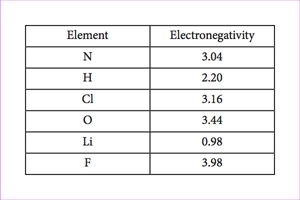Biology for AP® Courses

# Review Questions

Biology for AP® CoursesReview Questions
1.
What are atoms that vary in the number of neutrons found in their nuclei called?
1. Ions
2. Isotopes
3. Isobars
4. Neutral atoms
2.
Potassium has an atomic number of 19. What is its electron configuration?
1. Shells 1 and 2 are full, and shell 3 has nine electrons.
2. Shells 1, 2, and 3 are full, and shell 4 has three electrons.
3. Shells 1, 2, and 3 are full, and shell 4 has one electron.
4. Shells 1, 2, and 3 are full, and no other electrons are present.
3.
Which type of bond exemplifies a weak chemical bond?
1. Covalent bond
2. Hydrogen bond
3. Ionic bond
4. Nonpolar covalent bond
4.
Which of the following statements is false?
1. Electrons are unequally shared in polar covalent bonds.
2. Electrons are equally shared in nonpolar covalent bonds.
3. Hydrogen bonds are weak bonds based on electrostatic forces.
4. Ionic bonds are generally stronger than covalent bonds.
5.
If xenon has an atomic number of $54$ and a mass number of $108$, how many neutrons does it have?
1. $27$
2. $54$
3. $100$
4. $108$
6.
What forms ionic bonds?
1. atoms that share electrons equally
2. atoms that share electrons unequally
3. ions with similar charges
4. ions with opposite charges
7.Based on the information provided, which of the following statements is correct?

1. In NH2, the nitrogen atom acquires a partial positive charge and the hydrogen atoms acquire a partial negative charge.
2. In H2O, the hydrogen atoms acquire a partial negative charge, and the oxygen atom acquires a partial positive charge.
3. In aqueous HCl, the hydrogen atom acquires a partial positive charge, and the chlorine atom acquires a partial negative charge.
4. In LiF, the lithium atom acquires a positive charge, and the fluorine atom acquires a negative charge.
8.
Which of the following statements is not true?
1. Water is polar.
2. Water can stabilize the temperature of nearby air.
3. Water is essential for life.
4. Water is the most abundant molecule in the Earth’s atmosphere.
9.
Why do hydrogen and oxygen form polar covalent bonds within water molecules?
1. Hydrogen is more electronegative than oxygen, generating a partial negative charge near the hydrogen atom.
2. Hydrogen is more electronegative than oxygen, generating a partial positive charge near the hydrogen atom.
3. Oxygen is more electronegative than hydrogen, generating a partial negative charge near the oxygen atoms.
4. Oxygen is more electronegative than hydrogen, generating a partial positive charge near the oxygen atoms.
10.
What happens to the pH of a solution when acids are added?
1. The pH of the solution decreases.
2. The pH of the solution increases.
3. The pH of the solution increases and then decreases.
4. The pH of the solution stays the same.
11.
Which of the following statements is true?
1. Acids and bases cannot mix together.
2. Acids and bases can neutralize each other.
3. Acids, not bases, can change the pH of a solution.
4. Acids donate hydroxide ions ($OH Superscript minus$); bases donate hydrogen ions ($upper H Superscript plus$).
12.
1. a force that allows surface water molecules to escape and vaporize
2. the attraction between water molecules and other molecules
3. the intermolecular force between water molecules
4. the force that keeps particles dispersed in water
13.
In a solution, what kind of molecule binds up excess hydrogen ions?
1. acid
2. base
3. donator
4. isotope
14.
What is the maximum number of atoms or molecules a single carbon atom can bond with?
1. 4
2. 1
3. 6
4. 2
15.
Which of the following statements is true?
1. Molecules with the formulas $CH Subscript 3 Baseline CH Subscript 2 Baseline OH$ and $upper C Subscript 3 Baseline upper H Subscript 6 Baseline upper O Subscript 2$ could be structural isomers.
2. Molecules must have a single bond to be cis-trans isomers.
3. To be enantiomers, a molecule must have at least three different atoms or groups connected to a central carbon
4. To be enantiomers, a molecule must have at least four different atoms or groups connected to a central carbon
16.
Which of the following is not a functional group that can bond with carbon?
1. carbonyl
2. hydroxyl
3. phosphate
4. sodium
17.
Which of the following functional groups is not polar?
1. carbonyl
2. hydroxyl
3. methyl
4. sulfhydryl
18.
What are enantiomers?
1. Hydrocarbon consisting of closed rings of carbon atoms
2. Isomers with similar bonding patterns differing in the placement of atoms along a double covalent bond.
3. Molecules that share the same chemical bonds but are mirror images of each other.
4. Molecules with the same chemical formula but differ in the placement of their chemical bonds